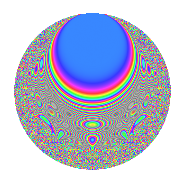# Properties

 Label 525.4.iLevel $525$ Weight $4$ Character orbit 525.i Rep. character $\chi_{525}(151,\cdot)$ Character field $\Q(\zeta_{3})$ Dimension $152$ Sturm bound $320$

# Related objects

## Defining parameters

 Level: $$N$$ $$=$$ $$525 = 3 \cdot 5^{2} \cdot 7$$ Weight: $$k$$ $$=$$ $$4$$ Character orbit: $$[\chi]$$ $$=$$ 525.i (of order $$3$$ and degree $$2$$) Character conductor: $$\operatorname{cond}(\chi)$$ $$=$$ $$7$$ Character field: $$\Q(\zeta_{3})$$ Sturm bound: $$320$$

## Dimensions

The following table gives the dimensions of various subspaces of $$M_{4}(525, [\chi])$$.

Total New Old
Modular forms 504 152 352
Cusp forms 456 152 304
Eisenstein series 48 0 48

## Trace form

 $$152q + 2q^{2} + 6q^{3} - 314q^{4} + 24q^{6} - 24q^{7} - 48q^{8} - 684q^{9} + O(q^{10})$$ $$152q + 2q^{2} + 6q^{3} - 314q^{4} + 24q^{6} - 24q^{7} - 48q^{8} - 684q^{9} - 20q^{11} + 72q^{12} - 4q^{13} + 322q^{14} - 1210q^{16} + 124q^{17} + 18q^{18} - 258q^{19} + 132q^{21} + 700q^{22} - 196q^{23} - 234q^{24} - 34q^{26} - 108q^{27} + 934q^{28} - 872q^{29} - 568q^{31} - 56q^{32} + 114q^{33} + 1752q^{34} + 5652q^{36} + 606q^{37} - 294q^{38} + 462q^{39} - 1208q^{41} - 408q^{42} - 396q^{43} - 20q^{44} + 1316q^{46} - 608q^{47} - 816q^{48} + 1080q^{49} + 228q^{51} + 1168q^{52} - 1396q^{53} - 108q^{54} - 4572q^{56} - 276q^{57} - 2554q^{58} + 1288q^{59} - 1908q^{61} + 2724q^{62} + 162q^{63} + 10612q^{64} + 156q^{66} - 770q^{67} + 5016q^{68} + 600q^{69} + 4688q^{71} + 216q^{72} + 2322q^{73} - 1910q^{74} + 7152q^{76} - 3108q^{77} - 1980q^{78} - 1772q^{79} - 6156q^{81} - 1288q^{82} + 296q^{83} - 3816q^{84} - 1646q^{86} + 1038q^{87} - 1438q^{88} + 3872q^{89} - 4594q^{91} + 7424q^{92} - 894q^{93} + 76q^{94} - 3354q^{96} + 396q^{97} + 9052q^{98} + 360q^{99} + O(q^{100})$$

## Decomposition of $$S_{4}^{\mathrm{new}}(525, [\chi])$$ into newform subspaces

The newforms in this space have not yet been added to the LMFDB.

## Decomposition of $$S_{4}^{\mathrm{old}}(525, [\chi])$$ into lower level spaces

$$S_{4}^{\mathrm{old}}(525, [\chi]) \cong$$ $$S_{4}^{\mathrm{new}}(7, [\chi])$$$$^{\oplus 6}$$$$\oplus$$$$S_{4}^{\mathrm{new}}(21, [\chi])$$$$^{\oplus 3}$$$$\oplus$$$$S_{4}^{\mathrm{new}}(35, [\chi])$$$$^{\oplus 4}$$$$\oplus$$$$S_{4}^{\mathrm{new}}(105, [\chi])$$$$^{\oplus 2}$$$$\oplus$$$$S_{4}^{\mathrm{new}}(175, [\chi])$$$$^{\oplus 2}$$

## Hecke characteristic polynomials

There are no characteristic polynomials of Hecke operators in the database